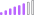cancel
Showing results for
Did you mean:Helper III

## Measure Help - Average Hours a Week Grouped into Buckets

I have the following table with the following columns

Time:

Emp ID | Date |  Week | # of Hours Charged

Data goes back 10 weeks

Week =Time[Date] - weekday(Time[Date],2) +1

Ive been asked to create a dynamic filter that would bucket the average number of hours someone charges by week:

Group 1 = Average 40 hours in a week

Group 2 = average Charging > 30 hours and <= 40 hours

Group 3 = average >20 hours && <=30 hours

Group 4 = average >10 hours && <= 20 hours

Group 5 = average <=10 hours

Currently the data is being displayed as follows:

Emp ID | Week 1 | Week 2 ...... Week 10

Emp A | 40 | 35 | 25 ... 10 - Averaging 35 hours a week

Emp B | 10 | 30 | 45 ... 50 - Averaging 30 hours a week

Does anyone know how to write this in DAX?

4 REPLIES 4New Member

Here is Switch conditon to use also if we need any kind of week like 7 days or 10 days bucket link in our report then we are used  only rhis dax.

First we make days in our Date field then we calculate the all of weeks count to sort of data field.Community Support

I solved the similar case here: https://community.powerbi.com/t5/Desktop/Chart-based-on-measure-buckets/td-p/795974

If this post helps, then please consider Accept it as the solution to help the other members find it.Post Patron
Below is a formula I used for my own buckets. I think if you adapt it to your data you should get what you need. Before you use it though just create a measure that takes the average of the hours charged and use that as the field being put into buckets. Let me know if this helps!

Usage_Buckets = if(YRMO_USAGE[BI_USAGE] <= 20000, "10,000 - 20,000", if(YRMO_USAGE[BI_USAGE] <= 40000, "20,001 - 40,000", if( YRMO_USAGE[BI_USAGE] <= 60000, "40,001 - 60,000", "60,000+")))Helper III

All of this makes sense with the buckets, but how do I make sure those buckets are calculating the weekly average hours? What calculation do I use to make sure the average measure is the average hours in a week (not a day, or by row in the table).